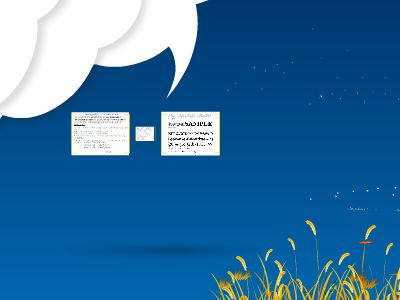Publications: 97 | Followers: 0

## Average atomic mass worksheet - Quia

Publish on Category: Birds 0

Average atomic mass worksheet
#1: Rubidium has two common isotopes,85Rb and87Rb.If the abundance of85Rb is 72.2% and the abundance of87Rb is 27.8%, what is the average atomic mass of rubidium?
PROCEDURE:1. Convert the 1stisotope’s percentage to a decimal (move decimal 2 places to the left)
72.2% → .722;
2. Multiply the decimal by the mass # for the isotope
.722x 85 =61.37
3. Write the product down; this is aSUB-TOTAL
4. Repeat steps 1-3 for each of the remaining isotopes listed, recording allSUB-TOTALS
27.8% → .278;
.278x 87 =24.186
5. Add your sub-totals together, and round to the lowest decimal place of the 2 subtotals -THIS IS THE ATOMIC MASS!
+

85.56
Average atomic mass worksheet
#2: Uranium has three common isotopes. If the abundance of234U is 0.01%, the abundance of235U is 0.71% and the abundance of238U is 99.28%, what is the average atomic mass of Uranium?
1. Convert the 1stisotope’s percentage to a decimal (move decimal 2 places to the left)
0.01% → .0001;
2. Multiply the decimal by the mass # for the isotope
.0001x 234 =.0234
3. Write the product down; this is aSUB-TOTAL
4. Repeat steps 1-3 for each of the remaining isotopes listed, recording allSUB-TOTALS
.71% → .0071;
.0071x 235 =1.6685
5. Add your sub-totals together, and round to the lowest decimal place of the 2 subtotals -THIS IS THE ATOMIC MASS!
+

=237.9783
99.28% → .9928;
.9928x 238 =236.2864
USE YOUR “READING THE PERIODIC TABLE: ….” NOTES – BOTTOM OF FRONT SIDE OF NOTEPAGE-IF YOU NEED GUIDANCE WITH THE MATH:

0

Embed

Share